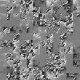author Amos RobinsonMon, 3 Dec 2012 04:46:00 +0000 (15:46 +1100) committer Amos RobinsonMon, 3 Dec 2012 04:46:00 +0000 (15:46 +1100)
 dph-examples/examples/spectral/Pluecker/Common.hs [new file with mode: 0644] patch | blob dph-examples/examples/spectral/Pluecker/CommonVectorised.hs [new file with mode: 0644] patch | blob dph-examples/examples/spectral/Pluecker/MainGloss.hs [new file with mode: 0644] patch | blob dph-examples/examples/spectral/Pluecker/Solver.hs [new file with mode: 0644] patch | blob dph-examples/examples/spectral/Pluecker/Vector.hs [new file with mode: 0644] patch | blob dph-examples/examples/spectral/Pluecker/Vectorised.hs [new file with mode: 0644] patch | blob

diff --git a/dph-examples/examples/spectral/Pluecker/Common.hs b/dph-examples/examples/spectral/Pluecker/Common.hs
new file mode 100644 (file)
index 0000000..599b564
--- /dev/null
@@ -0,0 +1,98 @@
+module Common where
+
+-- Type synonyms so they work with Data.Vector.Unboxed etc.
+
+-- A 3-vector *or* points
+type Vec3     = (Double, Double, Double)
+-- Line or ray between two points in space
+-- These are both points, so direction of (a,b) is the vector (b-a)
+type Line     = (Vec3, Vec3)
+
+-- Pl\"{u}cker coordinates of a line.
+-- To convert line (a,b) into pluecker, (b-a, a X b)
+type Pluecker = (Vec3,Vec3)
+
+-- Triangles are cool because they're obviously always planar.
+type Triangle = (Vec3, Vec3, Vec3)
+
+-- Normal and distance of normal from (0,0,0)
+type Plane = (Vec3,Double)
+
+
+{-# INLINE dot #-}
+dot :: Vec3 -> Vec3 -> Double
+dot (u,v,w) (x,y,z) = u*x + v*y + w*z
+
+{-# INLINE cross #-}
+cross :: Vec3 -> Vec3 -> Vec3
+cross (u,v,w) (x,y,z) = ( v * z - w * y
+                        , w * x - u * z
+                        , u * y - v * x)
+
+{-# INLINE over1 #-}
+over1 :: (Double -> Double) -> Vec3 -> Vec3
+over1 f (x,y,z) = (f x, f y, f z)
+
+{-# INLINE over2 #-}
+over2 :: (Double -> Double -> Double) -> Vec3 -> Vec3 -> Vec3
+over2 f (u,v,w) (x,y,z) = (f u x, f v y, f w z)
+
+{-# INLINE mag #-}
+mag a = sqrt (a `dot` a)
+
+{-# INLINE norm #-}
+norm a = over1 (/m) a
+ where m = mag a
+
+{-# INLINE vsub #-}
+vadd = over2 (+)
+vsub = over2 (-)
+
+-- Convert a line into pluecker coordinates
+{-# INLINE plueckerOfLine #-}
+plueckerOfLine :: Line -> Pluecker
+plueckerOfLine (p,q) = (q `vsub` p, q `cross` p)
+
+-- Find intersection of a line and plucker, if exists.
+-- Otherwise
+{-# INLINE projectPluecker2 #-}
+projectPluecker2 :: Pluecker -> Pluecker -> Double
+projectPluecker2 (u1,v1) (u2,v2) = (u1 `dot` v2) + (u2 `dot` v1)
+
+-- Check whether pluecker line intersects given triangle
+{-# INLINE inside #-}
+inside :: Pluecker -> Triangle -> Bool
+inside p (a,b,c)
+ = let pr = projectPluecker2 p . plueckerOfLine
+       ab = pr (a,b) < 0
+       bc = pr (b,c) < 0
+       ca = pr (c,a) < 0
+   in  (ab && bc && ca) || not (ab || bc || ca)
+
+
+-- Get plane from triangle, eg for projection
+{-# INLINE planeOfTriangle #-}
+planeOfTriangle :: Triangle -> Plane
+planeOfTriangle (a,b,c)
+ = let n  = (a `vsub` b) `cross` (c `vsub` b)
+       n' = norm n
+       d  = negate (n' `dot` b)
+   in  (n', d)
+
+-- should this return Maybe Double?
+{-# INLINE lineOnPlane #-}
+lineOnPlane :: Line -> Plane -> Double
+lineOnPlane (p,q) (n,d)
+ = let d1 = (n `dot` p) + d
+       d2 = (n `dot` q) + d
+   in  if   d1 == d2
+       then 1e100 -- meh. big. fail.
+       else d1 / (d1 - d2)
+
+-- Project line onto triangle's plane
+-- Disregard whether intersection is actually inside triangle
+-- Intersection point is scale from line
+{-# INLINE lineOnTriangle #-}
+lineOnTriangle :: Line -> Triangle -> Double
+lineOnTriangle p t
+ = lineOnPlane p (planeOfTriangle t)
diff --git a/dph-examples/examples/spectral/Pluecker/CommonVectorised.hs b/dph-examples/examples/spectral/Pluecker/CommonVectorised.hs
new file mode 100644 (file)
--- /dev/null
@@ -0,0 +1,103 @@
+{-# OPTIONS -fvectorise #-}
+module CommonVectorised where
+import Data.Array.Parallel.Prelude.Bool
+import Data.Array.Parallel.Prelude.Double        as D hiding (pi)
+import qualified Prelude    as P
+
+-- Type synonyms so they work with Data.Vector.Unboxed etc.
+
+-- A 3-vector *or* points
+type Vec3     = (Double, Double, Double)
+-- Line or ray between two points in space
+-- These are both points, so direction of (a,b) is the vector (b-a)
+type Line     = (Vec3, Vec3)
+
+-- Pl\"{u}cker coordinates of a line.
+-- To convert line (a,b) into pluecker, (b-a, a X b)
+type Pluecker = (Vec3,Vec3)
+
+-- Triangles are cool because they're obviously always planar.
+type Triangle = (Vec3, Vec3, Vec3)
+
+-- Normal and distance of normal from (0,0,0)
+type Plane = (Vec3,Double)
+
+
+{-# INLINE dot #-}
+dot :: Vec3 -> Vec3 -> Double
+dot (u,v,w) (x,y,z) = u*x + v*y + w*z
+
+{-# INLINE cross #-}
+cross :: Vec3 -> Vec3 -> Vec3
+cross (u,v,w) (x,y,z) = ( v * z - w * y
+                        , w * x - u * z
+                        , u * y - v * x)
+
+{-# INLINE over1 #-}
+over1 :: (Double -> Double) -> Vec3 -> Vec3
+over1 f (x,y,z) = (f x, f y, f z)
+
+{-# INLINE over2 #-}
+over2 :: (Double -> Double -> Double) -> Vec3 -> Vec3 -> Vec3
+over2 f (u,v,w) (x,y,z) = (f u x, f v y, f w z)
+
+{-# INLINE mag #-}
+mag a = sqrt (a `dot` a)
+
+{-# INLINE norm #-}
+norm a = over1 (/m) a
+ where m = mag a
+
+{-# INLINE vsub #-}
+vadd = over2 (+)
+vsub = over2 (-)
+
+-- Convert a line into pluecker coordinates
+{-# INLINE plueckerOfLine #-}
+plueckerOfLine :: Line -> Pluecker
+plueckerOfLine (p,q) = (q `vsub` p, q `cross` p)
+
+-- Find intersection of a line and plucker, if exists.
+-- Otherwise
+{-# INLINE projectPluecker2 #-}
+projectPluecker2 :: Pluecker -> Pluecker -> Double
+projectPluecker2 (u1,v1) (u2,v2) = (u1 `dot` v2) + (u2 `dot` v1)
+
+-- Check whether pluecker line intersects given triangle
+{-# INLINE inside #-}
+inside :: Pluecker -> Triangle -> Bool
+inside p (a,b,c)
+ = let pr l = projectPluecker2 p (plueckerOfLine l)
+       ab = pr (a,b) < 0
+       bc = pr (b,c) < 0
+       ca = pr (c,a) < 0
+   in  (ab && bc && ca) || not (ab || bc || ca)
+
+
+-- Get plane from triangle, eg for projection
+{-# INLINE planeOfTriangle #-}
+planeOfTriangle :: Triangle -> Plane
+planeOfTriangle (a,b,c)
+ = let n  = (a `vsub` b) `cross` (c `vsub` b)
+       n' = norm n
+       d  = negate (n' `dot` b)
+   in  (n', d)
+
+-- should this return Maybe Double?
+{-# INLINE lineOnPlane #-}
+lineOnPlane :: Line -> Plane -> Double
+lineOnPlane (p,q) (n,d)
+ = let d1 = (n `dot` p) + d
+       d2 = (n `dot` q) + d
+   in  if   d1 == d2
+       then 1e100 -- meh. big. fail.
+       else d1 / (d1 - d2)
+
+-- Project line onto triangle's plane
+-- Disregard whether intersection is actually inside triangle
+-- Intersection point is scale from line
+{-# INLINE lineOnTriangle #-}
+lineOnTriangle :: Line -> Triangle -> Double
+lineOnTriangle p t
+ = lineOnPlane p (planeOfTriangle t)
+
diff --git a/dph-examples/examples/spectral/Pluecker/MainGloss.hs b/dph-examples/examples/spectral/Pluecker/MainGloss.hs
new file mode 100644 (file)
index 0000000..9845406
--- /dev/null
@@ -0,0 +1,95 @@
+{-# LANGUAGE BangPatterns #-}
+
+import Common
+import Solver
+
+import Graphics.Gloss
+import Graphics.Gloss.Interface.IO.Animate
+
+import System.Environment
+import Data.Maybe
+import qualified Data.Vector                    as V
+import qualified Data.Vector.Unboxed            as VU
+
+import System.Random
+
+model :: Int -> Int -> IO (VU.Vector Vec3, VU.Vector (Int,Int,Int))
+model numverts numtris
+ = do
+   vs <- VU.generateM numverts mkvec
+   ts <- VU.generateM numtris  mktri
+   return (vs,ts)
+ where
+   mkvec _ = do
+     x <- randomRIO (-30,30)
+     y <- randomRIO (-30,30)
+     z <- randomRIO (0,10)
+     return (x,y,z)
+   mktri _ = do
+     a <- randomRIO (0,numverts - 1)
+     b <- randomRIO (0,numverts - 1)
+     c <- randomRIO (0,numverts - 1)
+     return (a,b,c)
+
+
+main :: IO ()
+main
+ = do   args    <- getArgs
+        mainWithArgs args
+
+
+mainWithArgs :: [String] -> IO ()
+mainWithArgs [solverName,numv,numt,numr]
+ = let  -- The solver we're using to calculate the acclerations.
+        solver      = fromMaybe (error \$ unlines
+                                        [ "unknown solver " ++ show solverName
+                                        , "choose one of "  ++ (show \$ map fst solvers) ])
+                        \$ lookup solverName solvers
+    in do
+        (v,t)   <- model (read numv) (read numt)
+        --let v = VU.fromList [(0,0,0),(1,1,1),(1,0,1)]
+        --let t = VU.fromList [(0,1,2)]
+        mainGloss v t (read numr) solver 400
+
+mainWithArgs [solverName] = mainWithArgs [solverName,"100","100","1000"]
+
+mainWithArgs _ = putStrLn "Usage: pluecker <vector|vectorised> <verts=100> <tris=100> <rays=1000>"
+
+
+-- | Run the simulation in a gloss window.
+mainGloss
+        :: VU.Vector Vec3
+        -> VU.Vector (Int,Int,Int)
+        -> Int          -- ^ number of rays
+        -> Solver       -- ^ Fn to calculate accels of each point.
+        -> Int          -- ^ Size of window.
+        -> IO ()
+
+mainGloss v t numr solver windowSize
+ = let  mkray _
+         = do   x <- randomRIO (-10,10)
+                y <- randomRIO (-10,10)
+                return (x,y,1)
+
+        draw _
+         = do   rays <- VU.generateM numr mkray
+                let pts = solver v t rays
+                return \$ Pictures \$ map drawPoint \$ VU.toList pts
+
+   in   animateIO
+                (InWindow  "Silly"                    -- window name
+                           (windowSize, windowSize)   -- window size
+                           (10, 10))                  -- window position
+                black                                 -- background color
+                draw                                  -- fn to convert a world to a picture
+
+
+
+pointSize = 20
+
+drawPoint :: (Vec3, Double) -> Picture
+drawPoint ((x,y,z), d)
+       = Translate (realToFrac x * 50) (realToFrac y * 50)
+    \$ Color (makeColor d' d' d' 1)
+       \$ ThickCircle (pointSize / 2) pointSize
+ where d' = 1 - (realToFrac \$ d / 10)
diff --git a/dph-examples/examples/spectral/Pluecker/Solver.hs b/dph-examples/examples/spectral/Pluecker/Solver.hs
new file mode 100644 (file)
index 0000000..0a17a8a
--- /dev/null
@@ -0,0 +1,23 @@
+module Solver
+    (Solver,solvers)
+where
+
+import Common
+import qualified Vector                         as SV
+import qualified Vectorised                     as SPA
+import qualified Data.Vector                    as V
+import qualified Data.Vector.Unboxed            as VU
+import qualified Data.Array.Parallel   as P
+import qualified Data.Array.Parallel.PArray    as P
+
+
+type Solver = VU.Vector Vec3 -> VU.Vector (Int,Int,Int) -> VU.Vector Vec3 -> VU.Vector (Vec3, Double)
+
+solvers :: [(String,Solver)]
+solvers =
+ [("vectorised", solverPA)
+ ,("vector",     SV.solveV)]
+
+solverPA verts tris rays
+ = let fv a = P.fromVector (VU.convert a)
+   in  VU.convert (P.toVector (SPA.solvePA (fv verts) (fv tris) (fv rays)))
diff --git a/dph-examples/examples/spectral/Pluecker/Vector.hs b/dph-examples/examples/spectral/Pluecker/Vector.hs
new file mode 100644 (file)
index 0000000..d600ff2
--- /dev/null
@@ -0,0 +1,40 @@
+module Vector
+    (solveV)
+where
+
+import Common
+import Data.Vector.Unboxed
+import Prelude hiding (map,filter,minimum)
+
+
+{-# NOINLINE solveV #-}
+solveV
+    :: Vector Vec3             -- ^ vertices of the surface
+    -> Vector (Int,Int,Int)    -- ^ triangles, each 3 vertex indices
+    -> Vector Vec3             -- ^ rays to cast
+    -> Vector (Vec3,Double)    -- ^ rays and their distance
+solveV vertices triangles rays
+ = map cast' rays
+ where
+  cast' = cast vertices triangles
+
+
+cast
+    :: Vector Vec3          -- ^ vertices of the surface
+    -> Vector (Int,Int,Int) -- ^ triangles, each 3 vertex indices
+    -> Vec3                 -- ^ ray
+    -> (Vec3,Double)
+cast vertices triangles ray
+ = let r' = ((0,0,0), ray)
+       pl = plueckerOfLine r'
+       mi = minimum
+          \$ map (\t -> check r' pl \$ tri vertices t) triangles
+   in  (ray, mi)
+
+check r pl t
+  | inside pl t = lineOnTriangle r t
+  | otherwise = 1e100
+
+tri :: Vector Vec3 -> (Int,Int,Int) -> Triangle
+tri v (a,b,c) = (v ! a, v ! b, v ! c)
+
diff --git a/dph-examples/examples/spectral/Pluecker/Vectorised.hs b/dph-examples/examples/spectral/Pluecker/Vectorised.hs
new file mode 100644 (file)
index 0000000..29d4d77
--- /dev/null
@@ -0,0 +1,54 @@
+{-# LANGUAGE ParallelArrays, ParallelListComp #-}
+{-# OPTIONS -fvectorise #-}
+
+module Vectorised
+    (solvePA)
+where
+
+import CommonVectorised
+import Data.Array.Parallel hiding ((+), (-), (*), (/))
+import Data.Array.Parallel.PArray
+import Data.Array.Parallel.Prelude.Bool
+import Data.Array.Parallel.Prelude.Double        as D
+import qualified Data.Array.Parallel.Prelude.Int as I
+import qualified Prelude    as P
+
+
+{-# NOINLINE solvePA #-}
+solvePA
+    :: PArray Vec3           -- ^ vertices of the surface
+    -> PArray (Int,Int,Int)  -- ^ triangles, each 3 vertex indices
+    -> PArray Vec3           -- ^ rays to cast
+    -> PArray (Vec3,Double)  -- ^ rays and their distance
+solvePA vertices triangles rays
+ = toPArrayP (solveV (fromPArrayP vertices) (fromPArrayP triangles) (fromPArrayP rays))
+
+solveV
+    :: [:Vec3:]             -- ^ vertices of the surface
+    -> [:(Int,Int,Int):]    -- ^ triangles, each 3 vertex indices
+    -> [:Vec3:]             -- ^ rays to cast
+    -> [:(Vec3,Double):]    -- ^ rays and their distance
+solveV vertices triangles rays
+ = mapP cast' rays
+ where
+  cast' = cast vertices triangles
+
+
+cast
+    :: [:Vec3:]          -- ^ vertices of the surface
+    -> [:(Int,Int,Int):] -- ^ triangles, each 3 vertex indices
+    -> Vec3              -- ^ ray
+    -> (Vec3,Double)
+cast vertices triangles ray
+ = let r' = ((0,0,0), ray)
+       pl = plueckerOfLine r'
+       mi = minimumP
+            (mapP (\t -> check r' pl (tri vertices t)) triangles)
+   in  (ray, mi)
+
+check r pl t
+  | inside pl t = lineOnTriangle r t
+  | otherwise = 1e100
+
+tri :: [:Vec3:] -> (Int,Int,Int) -> Triangle
+tri v (a,b,c) = (v !: a, v !: b, v !: c)